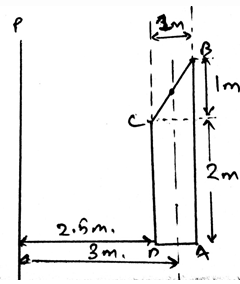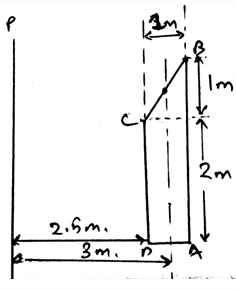Courses

# Test: Theorem Of Pappus And Guldinus

## 15 Questions MCQ Test Engineering Mechanics | Test: Theorem Of Pappus And Guldinus

Description
This mock test of Test: Theorem Of Pappus And Guldinus for Mechanical Engineering helps you for every Mechanical Engineering entrance exam. This contains 15 Multiple Choice Questions for Mechanical Engineering Test: Theorem Of Pappus And Guldinus (mcq) to study with solutions a complete question bank. The solved questions answers in this Test: Theorem Of Pappus And Guldinus quiz give you a good mix of easy questions and tough questions. Mechanical Engineering students definitely take this Test: Theorem Of Pappus And Guldinus exercise for a better result in the exam. You can find other Test: Theorem Of Pappus And Guldinus extra questions, long questions & short questions for Mechanical Engineering on EduRev as well by searching above.
QUESTION: 1

### The theorem of Pappus and Guldinus is used to find the ____________

Solution:

The theorem is used to find the surface area and the volume of the revolving body. This is done by using simple integration. Thus the surface area and the volume of any 2D curve being rotated can be made to be calculated from this theorem.

QUESTION: 2

### The theorem of Pappus and Guldinus states that the area of the revolving curve is ______________

Solution:

The theorem is used to find the surface area and the volume of the revolving body. This is done by using simple integration. Thus the surface area and the volume of any 2D curve. It is just the product of the area, length of generated curve and the perpendicular distance from axis.

QUESTION: 3

### The theorem of Pappus and Guldinus is used to find the ____________

Solution:

The theorem is used to find the surface area and the volume of the revolving body. This is done by using simple integration. Thus the surface area and the volume of any 2D curve being rotated can be made to be calculated from this theorem.

QUESTION: 4

Determine the surface area of the structure when rotated about the ray P.Solution:

The theorem is used to find the surface area and the volume of the revolving body. This is done by using simple integration. Thus the surface area and the volume of any 2D curve. It is just the product of the area, length of generated curve and the perpendicular distance from axis.

QUESTION: 5

Product of the area, ______________________ and the perpendicular distance from axis are being used in the theorem to find the area of the revolution.

Solution:

The theorem is used to find the surface area and the volume of the revolving body. This is done by using simple integration. Thus the surface area and the volume of any 2D curve. It is just the product of the area, length of generated curve and the perpendicular distance from axis.

QUESTION: 6

The distance used in the theorem is the distance travelled by ______________

Solution:

The theorem is used to find the surface area and the volume of the revolving body. Thus the surface area and the volume of any 2D curve. It is just the product of the area, length of generated curve and the perpendicular distance from axis. The distance being the distance travelled by the centroid.

QUESTION: 7

Product of the Volume, ______________________ and the perpendicular distance from axis are being used in the theorem to find the volume of the revolution.

Solution:

The theorem is used to find the surface area and the volume of the revolving body. This is done by using simple integration. Thus the surface area and the volume of any 2D curve. It is just the product of the volume, area of generated curve and the perpendicular distance from axis.

QUESTION: 8

The maximum value of the frictional force that can be applied to the composite body under which the theorem of the Pappus and Guldinus is going to be applied is called _________

Solution:

The friction is the phenomena that defines that there is a resistance which is present there between the two surfaces. The two surfaces are in contact and the friction applies at that surface only, resisting the motion of the surface. Thus the maximum values is called as limiting friction.

QUESTION: 9

Determine the volume of the structure when rotated about the ray P.Solution:

The theorem is used to find the surface area and the volume of the revolving body. This is done by using simple integration. Thus the surface area and the volume of any 2D curve. It is just the product of the area, length of generated curve and the perpendicular distance from axis.

QUESTION: 10

When the body which is applied forces come in the stage of the limiting friction then the body over which this theorem is to be applied is termed as to come in ___________ equilibrium.

Solution:

As the limiting friction is the maximum value of the frictional forces. Thus if more force is applied to the body then the body is going to move forward. Because the two surfaces are in contact and the friction applies at that surface only, resisting the motion of the surface. Thus the name unstable equilibrium.

QUESTION: 11

We use sometimes the measures to know the direction of moment. It is done by right handed coordinate system. Which is right about it for the bodies over which this theorem is going to be applied (consider the mentioned axis to be positive)?

Solution:

As right handed coordinate system means that you are curling your fingers from positive x-axis towards y-axis and the thumb which is projected is pointed to the positive z-axis. Thus visualizing the same and knowing the basic members of axis will not create much problem.

QUESTION: 12

What is not the condition for the equilibrium in three dimensional system of axis for the bodies for which this theorem is going to be applied?

Solution:

For the equilibrium in the three dimensional system of axis we have all the conditions true as, ∑Fx=0, ∑Fy=0 and ∑Fz=0. Also we have the summation of the forces equal to zero. Which is not a non-zero value.

QUESTION: 13

What is a collinear system of forces for the bodies over which this theorem is to apply?

Solution:

The force system having all the forces emerging from a point is called the collinear system of force. This is a type of system of the force, which is easy in the simplification. This is because as the forces are the vector quantity, the vector math is applied and the simplification is done.

QUESTION: 14

The system of the collinear and the parallel force for the bodies over which this theorem is to be applied are simplified as:

Solution:

The simplification is easy. The simplified force system gives us a net force and the parallel force system gives us a simplified force, and then we add it vectorially. Thus the vector addition at the last can be easily done and thus both can be simplified.

QUESTION: 15

If solving the question in 3D calculations is difficult, then use the 2D system to make the use of the theorem.

Solution:

The answer is obviously yes. If we are having any difficulty in making the vector components, then we can go in 2D. As if the particle is in equilibrium, the net force will be zero. No matter where you see first. Net force is zero.